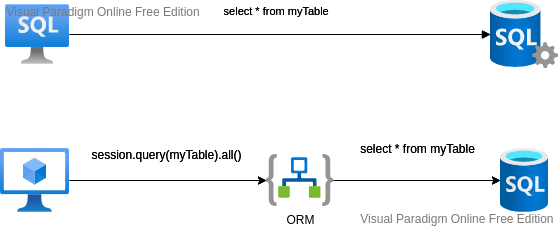#DAY 26
0

## 資料儲存 - SqlAlchemy1. 安裝 SqlAlchemy
``````pip install sqlalchemy
``````

sqlite 有內建的 DB-API，不需要另外安裝

1. 建立資料庫連接類別

database.py

``````from sqlalchemy import create_engine
from sqlahchemy.ext.declarative import declarative_base
from sqlalchemy.orm import sessionmaker

SQLALCHEMY_DATABASE_URL = "sqlite:///stock.db"

engine = create_engine(
)

session = ssessionmaker(autocommit=False, autoflush=False, bind=engine)

Base = declarative_base()

``````

1. 建立 model 類別

models.py

``````
from sqlalchemy import Column, String, DateTime, DECIMAL, Integer
from .database import Base

class Kbars(Base):
__tablename__ = 'kbars'

stock_code = Column(String, primary_key=True)
datetime = Column(DateTime(timezone=False), primary_key=True)
open = Column(DECIMAL(precision=2))
high = Column(DECIMAL(precision=2))
low = Column(DECIMAL(precision=2))
close = Column(DECIMAL(precision=2))
volume = Column(Integer)

def __init__(self, stock_code, datetime, open, high, low, close, volume):
self.stock_code = stock_code
self.datetime = datetime
self.open = open
self.high = high
self.low = low
self.close = close
self.volume = volume

def __repr__(self):
return "Stock: {} datetime: {} open:{}|high:{}|low:{}|close:{}|volume:{}".format(self.stock_code, self.datetime, self.open .self.high, self.low, self.close, self.volume)
``````
1. 呼叫 sqlalchemy：
基本上相關的設定就在上面了，接下來就是在我們要存取資料的地方，呼叫 sqlalchemy

main.py

``````from models import Kbars
from sqlalchemy.sql.expression import and_
from database import session, engine
from sqlalchemy import func
from datetime import datetime

db = session()

# 這一段很重要，會自動建立 table

# 新增一筆假資料
kbar = Kbar("2330", datetime(2021, 10, 4, 13, 17), 571, 571, 570, 570, 129)

db.commit()

kbars = db.query(Kbars)\
.filter(and_(func.date(Kbars.datetime) >= start,
func.date(Kbars.datetime) <= end,
Kbars.stock_code == stock_code)).all()
``````